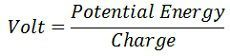# Difference Between Volts & Watts

One of the major difference between volts and watts is that the volt is the SI unit of potential difference and electromotive force, whereas the watt measures the SI unit of power. The other differences between them are explained below in the comparison chart.

Both the volts and watts are related to each other. The volt measures the potential differences of supply sources or the voltage of the electrical devices. The symbolic representation of the volt is V. The measurement taken in volts is easier as compared to watts because watts is the product of the two quantity i.e., voltage and current. The watt is represented by W. It measures the power used by the electric devices.

## Comparison Chart

Basis for Comparison VoltsWatts
Definition It is the SI unit of potential difference and EMF.It is the SI unit of Power.
FormulaUnits of Electromotive force and potential difference Power
Symbol V W
Measurement Measure small amount of voltage from power supply.Measures realistic power.
Measuring Device Voltmeter Power Meter
Base Unit KgM2S-3KgM2A-1S-3

## Definition of Volt

The volts measure the energy utilise by the electrons moving from one end to another. Symbolically it is represented by the capital alphabetical letter V. It is measured by the electrical instrument called a voltmeter. The volt has various subunits like micro-volt, millivolt, kilovolt, etc.

## Definition of Watt

The watt is the SI unit of power. It is defined as the total energy utilised by the devices in one second. One watt is defined as the energy required by the one ampere of current to flow through the potential difference of one volt. The power is the product of the voltage and current, thus for measuring the power in watts both volt and amp requires.

### Key Differences Between Volts and Watts

1. The volt is the SI unit of electromotive force and potential differences, whereas the watt is the SI unit of power.
2. The symbolic representation of volts is V whereas the watt is represented by the symbol W.
3. The reading takings in volt are easier as compared to watts because watt requires both the quantities of voltage and current.
4. In volts, a small amount of power is measured whereas watt measure the real power utilises by the electrical devices.
5. The value in volts is measured by the voltmeter whereas watt is measured by the power meter.
6. The base unit of watts is KgM2S-3 and the base unit of a volt is KgM2A-1S-3. The base unit is the fundamental unit which is not combined with any other unit examples – meter, kilogram, second, ampere etc.

The international standard unit is globally accepted for measurement.